Arc-Sided Figures
 To the Main Page    "Mathematische Basteleien"

What are Arc-Sided Figures?

Arc-sided figures are figures, which are formed by (circular) arcs.

This section contains a collection of arc figures arranged by the number of corners. On this web page a corner is the point, where two arcs touch.The colours of the figures mark the symmetry.

1 No symmetry
 2 symmetry with 1 centre 3 symmetry with 1 axis 4 symmetry with 2 axes  5 symmetry with 3 axes 6 symmetry with 4 axes  7 symmetry with 5 axes and more

The letters below the following figures mean: A = area, U = circumference.

Figures of two circles    top
 Lense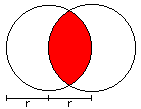A = [2/3*Pi - 1/2*sqrt(3)]*r² U = 4/3*Pi*r Lense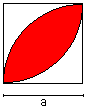A =  [1/2*Pi - 1]*a² U = Pi*a MushroomA = (1/8*Pi - 1/4]*r² U = 1/2*Pi*r

Three-quarter Moon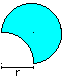A = [1/2*Pi + 1]*r²
U = 2*Pi*r

Figures of three circles   top
 Tricorn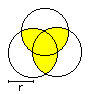A = [Pi - 1/2*sqrt(3)]*r² U=2*Pi*r Bug's Eyes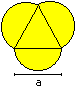A = [1/4*sqrt(3) + 3/8*Pi]*a² U = 3/2*Pi*a Three circlesA = [1/4*sqrt(3) + 5/8*Pi]*a² U = 5/2*Pi*a

 Arbelos, Shoemaker's Knife (Archimedes)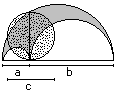A = 1/4*Pi*ab = 1/4*Pi*c² U = (a+b)*Pi Crescent Moons (Hippokrates)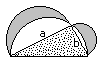A = 1/2*a*b U = Pi/2*[a+b+sqrt(a²+b²)] Ying und Yang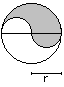A = 1/2*Pi*r² U = 2*Pi*r

 Spinning TopA = 2*r² U = 2*Pi*r Golf Tee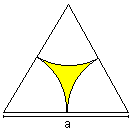A = [1/4*sqrt(3) - 1/8*Pi]*a² U = 1/2*Pi*a Arc TriangleA = [1/2*Pi - 1/2*sqrt(3)]*r² U = Pi*r

Figures of four circles        top
 Cruciferous Flower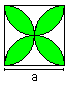A = (1/2*Pi - 1]*a² U = 2*Pi*a Orbital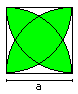A = [sqrt(3) + 2/3*Pi - 3]*a² U = 4/3*Pi*a Cross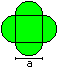A = [1 + 1/2*Pi]*a² U = 2*Pi*a

 Diamonds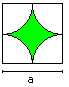A = (1 - Pi/4)*a² U = Pi*a Axe headA = 1/2*a² U = Pi*a Arc square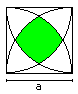A = [1 + 1/3*Pi - sqrt(3)]*a² U = 2/3*Pi*a

 Four-Leaf CloverA = [1 + 3/4*Pi]*a² U = 3*Pi*a Dumb-Bell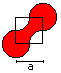A = [1 + 1/4*Pi]*a² U = 2*Pi*a Heart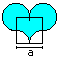A = [1 + 1/4*Pi]*a² U = 2*Pi*a

 HookA = 3/4*Pi*r² U = 3*Pi*r SalinonA = 1/4*PI*(a+b)² = 1/4*Pi*c² U = Pi*(2*a+b) Worm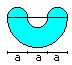A = 5/4*Pi*a² U = 3*Pi*a

 RingA = [1/24*Pi + 1/4*sqrt(3)]*a² U = [2/3*sqrt(3)+3/2]*Pi*a Hour-glass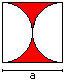A = [1 - 1/4*Pi]*a² U = Pi*a Chicken egg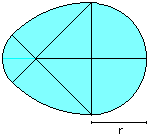A = [3*Pi - sqrt(2)*Pi - 1)]*r² U = [3-1/2*sqrt(2)]*Pi*r

Figures of five circles     top
 Crescent Moons (Hippokrates)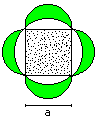A = a² U = [sqrt(2)*+2]*a TulipA=Pi*r² U = (2*Pi+2)*r

Figures of six circles   top
 Rosette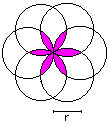A = [2*Pi - 3*sqrt(3)]*r² U = 4*Pi*r Rosette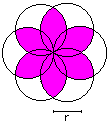A = 2*Pi *r² U = 4*Pi*r Bear headA = [1/4*sqrt(3) + 1/16*Pi]*a² U = Pi*a

 Curve with constant width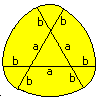A = 1/2*Pi*a² + Pi*ab + Pi*b² - 1/2*sqr(3)*a² U = Pi*(a+2b) Humming-Top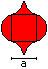A = 2*a² U = 2*Pi*a

Figures of eight circles      top
 Cross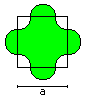A = [1 + 1/16*Pi]*a² U = 3/2*Pi*a Cross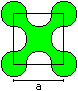A = [1 + 1/16*Pi]*a² U = 5/2*Pi*a

Rings    top
 Circumscribed and inscribed circle of  an equilateral triangle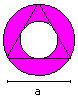A = 1/4*Pi*a² U = sqr(3)*Pi*a (more precise: boundary line) Circumscribed and inscribed circle of  a square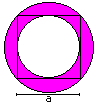A = 1/4*Pi*a² U = [1+sqrt(2)]*Pi*a (more precise: boundary line)

How to Calculate Arc-Sided Figures      top
You calculate arc-sided figures by searching basic figures, which form the figures and the areas of which you know. You must multiply, subtract, add them.

This method will be explained by three figures.

1st Example
The only basic figure is the semi-circle, that appears four times. The area is generally 1/2*Pi*r². You replace the radius r by a/2 and/or 3a/2.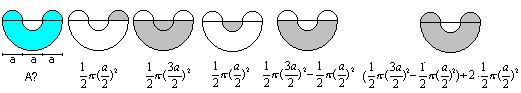The best way is to only write the term on the right and simplify it. The result is A=5/4*Pi*a².

2nd Example
The second figure consists of an equilateral triangle and three congruent arcs.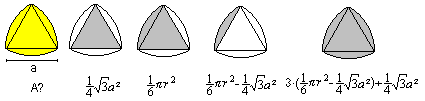The result is A = [1/2*Pi - 1/2*sqr(3)]*r².

3rd Example
The third figure is formed by two congruent arcs. The basic figures are a quarter circle and a half square.The result is A = [1/2*Pi - 1]*r².

 ...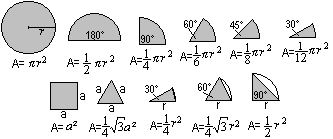. A collection of important basic figures

Some solutions  top
Area of the arc square
 ...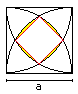The calculation has two parts: 1 You calculate the area of the inner red square. 2 You calculate the area of the yellow segments.

1
 ...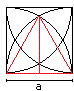...... There is a equilateral triangle inside the square with the height h=(1/2)sqrt(3)a. The blue lines are the diagonals of the square. One half diagonal has the length h-(1/2)a=(1/2)[sqrt(3)-1]a Thus the area of the square is A1 = 4*(1/2){(1/2)[sqrt(3)-1]a}² = (1/2)[4-2sqrt(3)]a²=[2-sqrt(3)]a².

2
 ...... There is a 30° sector inside the square.  The area of the segment is the difference between the area of the sector and the area of the isosceles triangle with the sides a. There are four segments. A2 = 4*[(1/12)pi*a²-(1/4)a²] = [(1/3)pi-1]a²

Result
A=A1 + A2 = [2-sqrt(3)]a² + [(1/3)pi-1]a² = [1-sqrt(3)+(1/3)pi]a²

Area of the OrbitalI use the formulas of the lense  AL =  [1/2*Pi - 1]*a² and  the arc square AB = [1 + 1/3*Pi - sqrt(3)]*a².The area of the orbital is A = 2*AL - AB. That means A=2*[1/2*Pi - 1]*a² -[1 + 1/3*Pi - sqrt(3)]*a² oder A = Pi*a²-2a²-a²-(1/3)Pi*a²+sqrt(3)a² oder  A = (2/3)Pi*a²-3a²+sqrt(3)a² oder A = [sqrt(3) + 2/3*Pi - 3]*a², qed.

Will be continued

Squaring the Circle top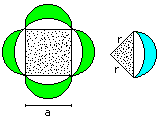In the history of mathematics Hippokrates' crescent moons were important, because you can draw a triangle (or four-sided figures in other cases) with ruler and compass having the same area. Mathematicians guessed you were able to find a square with the same area as a circle in a similar way. But since the 19th century it is known that this is not possible, because Pi is a transcendental number (Ferdinand Lindemann 1882).

The crescent moon figures are still interesting today, because five "constructable" arc figures with two corners are known. It is unknown, whether there are more.

You find more about circles on my German pages Kreis, Kreise im Kreis, Arbelos, Halbkreis, Zweikreisfiguren, and Gleichdick.

Arc-Sided Figures on the Internet   top

German

Barbara Flütsch (Mathe-Aufgaben)
Kreis und Kreisteile: Berechnungen

Wikipedia
Kreis (Geometrie)Reuleaux-Dreieck, Arbelos

English

David Eppstein    (The Geometry Junkyard)
Circles and Spheres

Eric W. Weisstein  (MathWorld)
Piecewise Circular Curve, Circle, Arc, Triangle Arcs, Semicircle, Arbelos, Lens
Yin-Yang, Salinon, Reuleaux Triangle

Tim Lexen
Bending the Law of Sines, Making Tricurves

University of Cambridge (nrich mathematics)

Wikipedia
Circle, Reuleaux triangle, Arbelos, Salinon, Semicircle, Ring (geometric)

References   top
Walter Lietzmann: Altes und Neues vom Kreis, Leipzig und Berlin 1935
Eugen Beutel: Die Quadratur des Kreises, Leipzig und Berlin 1942
Maximilian Miller: Gelöste und ungelöste mathematische Probleme, Leipzig 1973

10a in 1986/87, thank you
Gail from Oregon Coast, thank you for supporting me in my translation.

Feedback: Email address on my main page